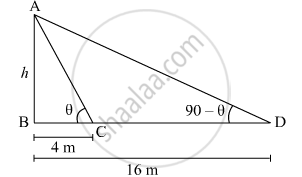Share

# On a Straight Line Passing Through the Foot of a Tower, Two Points C and D Are at Distances of 4 M and 16 M from the Foot Respectively. If the Angles of Elevation from C and D of the Top of the Tower Are Complementary, Then Find the Height of the Tower. - CBSE Class 10 - Mathematics

#### Question

On a straight line passing through the foot of a tower, two points C and D are at distances of 4 m and 16 m from the foot respectively. If the angles of elevation from C and D of the top of the tower are complementary, then find the height of the tower.

#### Solution

Let AB be the tower of height h m. Suppose the angle of elevation of the top of the tower from point C on the ground be θ. Then the angle of elevation of the top of the tower from point D is 90°− θ.

Here, BC = 4 m and BD = 16 mIn ΔABC

tan theta = (AB)/(BC) = h/4 .....(1)

In ΔABD

tan(90^@ - theta) = (AB)/(BD) = h/16

=> cot theta = h/16 .....(2)

Multiplying (1) and (2), we get

tan theta xx cot theta = h/4 xx h/16 = h^2/64

=> 1 = h^2/64

=> h^2 = 64

=> h = 8 m

Thus, the height of the tower is 8 m.

Is there an error in this question or solution?

#### Video TutorialsVIEW ALL 

Solution On a Straight Line Passing Through the Foot of a Tower, Two Points C and D Are at Distances of 4 M and 16 M from the Foot Respectively. If the Angles of Elevation from C and D of the Top of the Tower Are Complementary, Then Find the Height of the Tower. Concept: Heights and Distances.
S